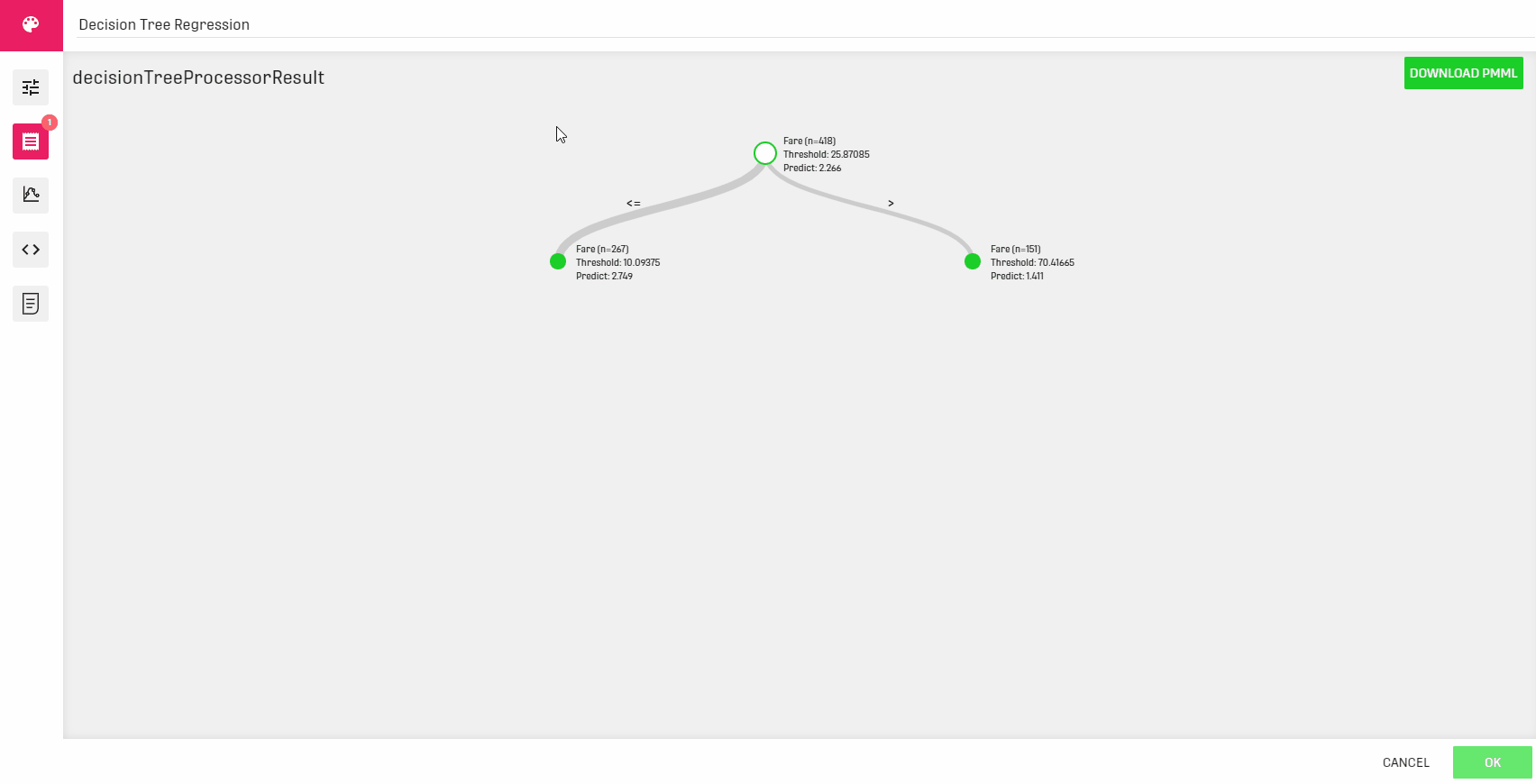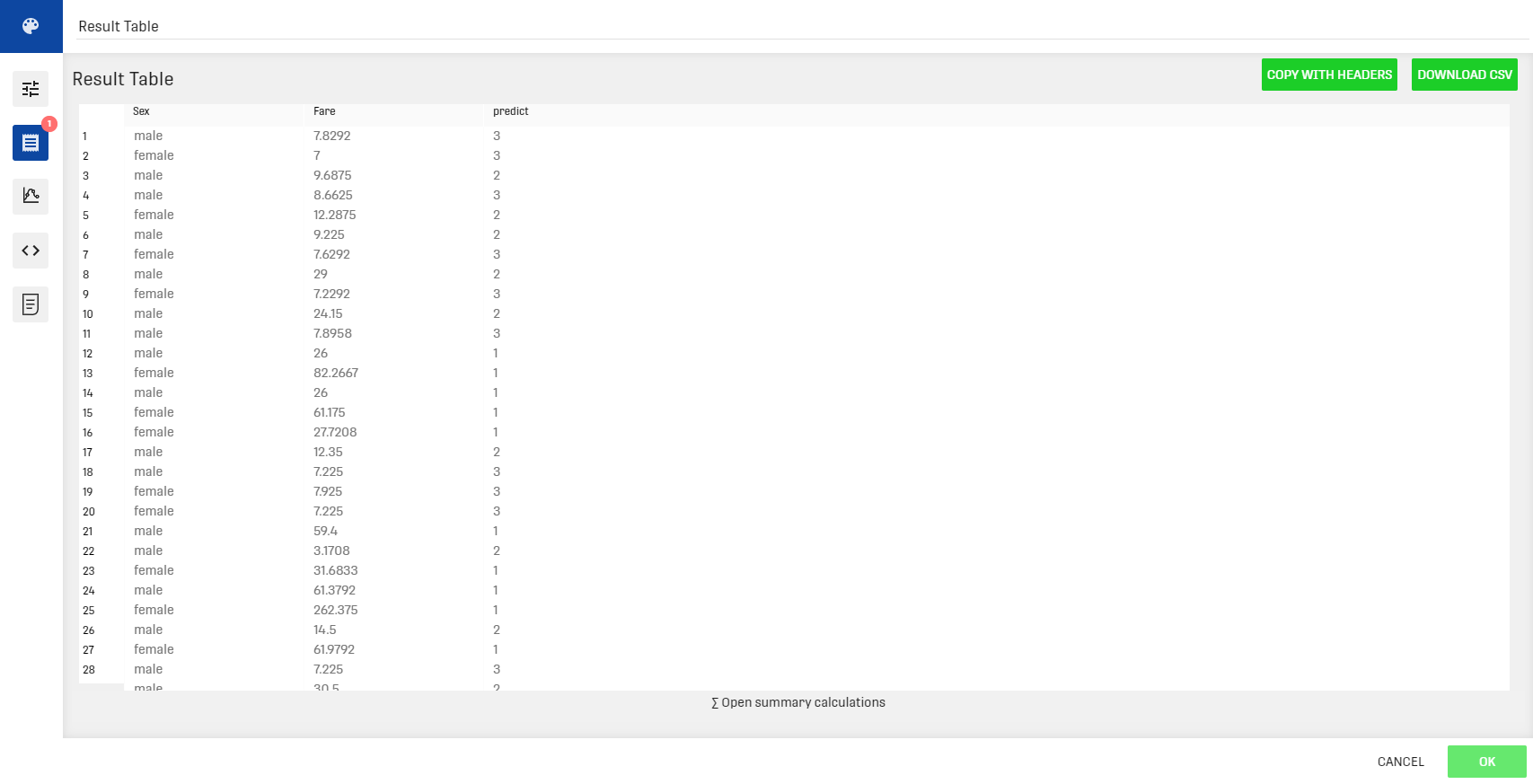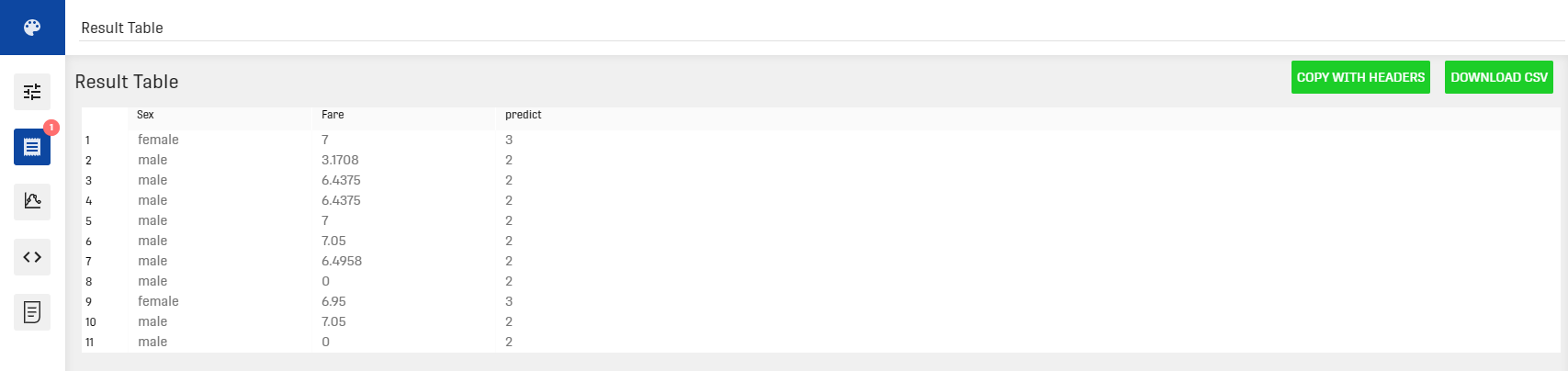# Overview

The Decision Tree Regression Processor creates a decision tree for regression out of the input dataset.

Regression is a statistical measurement that attempts to estimate the relationship between one dependent variable (usually denoted by Y) and a series of other changing variables (known as independent variables).

# Input

The processor works on any dataset that contains at least one numeric variable, that is to be the dependent variable (the one to estimate).

# Output

The Decision Tree Regression Processor provides two different outputs:

• A decision tree: this tree can be accessed through the decision tree classification processor under the "Results" tab. It can also be downloaded in XML format.
• A prediction table: a table presenting the different independent variables along with the predicted value for the dependent variable. It can be viewed via the result table linked to the processor's output.

Note that the prediction in the decision tree and in the output table may not be equal. This will be further explained by the following example. Also keep in mind that, just because no errors are shown, that doesn't mean the regression is reasonable. It depends on the choice of the independent variables.

# Example

In this example, the input dataset represents information about train passengers (Name, Class, Age...). The goal is to build a decision tree that estimates the passenger's travelling class using the fare and sex attributes.

## Result

Decision treeResult tableThe prediction is outputted in the added column named "predict" along with the specified independent variables.

Notice here that the prediction within the tree is a float number, that is the immediate result of the algorithm. However, in the result table it is converted to an integer, which is the desired format of the result.

For further explanation, here in the result tree, the passengers with a fare inferior to the value 7.13749999..

have the prediction 3.0 in case of a female passenger and the prediction 2.556 in case of a male passenger.

Now if we try to select only the passengers with the mentioned fare value (inferior to 7.13749999..) the result table will be as follows:In the second result table the prediction value 2.556 is rounded to 2 for male passengers.

# Related Articles

Decision Tree Classification Processor

Decision Tree Classification Forecast Processor

Decision Tree Regression Forecast Processor